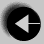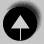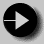## Using #define to Create Functional Macros

#define can also be given arguments which are used in its replacement. The definitions are then called macros. Macros work rather like functions, but with the following minor differences.

• Since macros are implemented as a textual substitution, there is no effect on program performance (as with functions).
• Recursive macros are generally not a good idea.
• Macros don't care about the type of their arguments. Hence macros are a good choice where we might want to operate on reals, integers or a mixture of the two. Programmers sometimes call such type flexibility polymorphism.
• Macros are generally fairly small.

Macros are full of traps for the unwary programmer. In particular the textual substitution means that arithmetic expressions are liable to be corrupted by the order of evaluation rules.

Here is an example of a macro which won't work.

```
#define DOUBLE(x) x+x```

Now if we have a statement

```
a = DOUBLE(b) * c;```

This will be expanded to

```
a = b+b * c;```

And since * has a higher priority than +, the compiler will treat it as.

```
a = b + (b * c);```

The problem can be solved using a more robust definition of DOUBLE

```
#define DOUBLE(x) (x+x)```

Here the brackets around the definition force the expression to be evaluated before any surrounding operators are applied. This should make the macro more reliable.

In general it is better to write a C function than risk using a macro.Go to Using #define (1)Go to IndexGo to Using #include